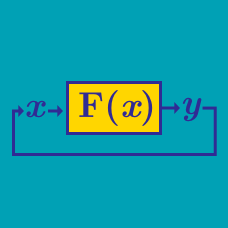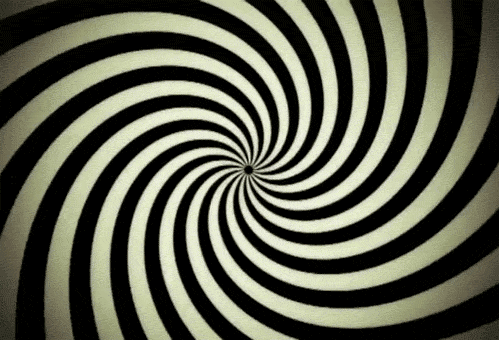Computer Science

# Functions (CS): Level 3 Challenges

You have in your wallet $300, which you want to spend completely. You decide to spend all of it by buying food from a fancy restaurant with the following menu:  tofu scramble:$1
pancakes: $5 brunch combo:$20
saffron infused peach tea: $50 truffles:$100
caviar: $200  Let $O$ be the number of different ways that you can spend exactly$300. What are the last 3 digits of $O$?

The number

$\huge{(1+\sqrt{2})^{27}}$

can be represented as $x\sqrt{y} + z$ where $x,y$ and $z$ are positive integers.Find $x+y+z$.

For all positive integers $n$, the totient function $\phi (n)$ denotes the number of positive integers $\leq n$ coprime to $n$.

It turns out that if we apply the totient function successively on any positive integer $n>1$, after finitely many operations we get the number $1$. In other words, for all positive integers $n>1$, there exists a positive integer $m$ such that $\underbrace{\phi ( \phi ( \phi ( \cdots \phi (n ) \cdots )))}_{m \text{ times}}= 1$.

For all $n \in \mathbb{N}$, let $f(n)$ denote the minimum number of times we need to apply the totient function successively on $n$ to get the number $1$. Find the last three digits of $\displaystyle \sum \limits_{n=1}^{2014} f(n)$.

Details and assumptions

• As an explicit example, here's how you'd find $f(5)$: $\begin{array}{rcl} \phi (5)& = & 4 \\ \phi (\phi (5)) & = & 2 \\ \phi (\phi (\phi (5))) & = & 1 \\ \end{array}$ Note that we had to apply the totient function three times on $5$ to get the number $1$, so $f(5)=3$.

• By convention, $f(1)=0$.

• Just to clarify, this is a computer science problem.Consider the rectangular spiral in the image below. It starts from the origin and twirls and twirls forever in an anticlockwise direction along the integer coordinates of the Cartesian coordinate plane.Each point along the spiral is numbered with an integer $Z$ as shown in the image below.What is the value of the integer $Z$ at the coordinate $(12,-22)$?

Details and assumptions

As an explicit example $Z$ is $10$ for $(2,0)$ , $3$ for $(0,1)$ and $10$ for $(2,0)$.

Given a list of integers $1\cdots n$, return the count of the number that have the number $k$ in them. For $n\leq10^{9}$ how many numbers have the number $7$ in them.

Details and Assumptions

For $n\leq10$, the number of occurrence of $7$ is one.

For $n\leq100$ the number of occurrence of $7$ is $19$...$7,17,27,37,47,57,67,70,71\cdots ,79,87,97$

×

Problem Loading...

Note Loading...

Set Loading...